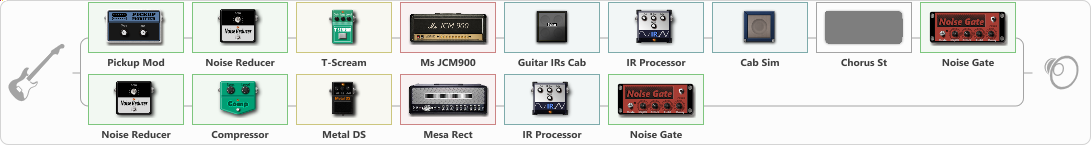# super palm-mute

Discussion in 'ToneLib-GFX presets' started by GangGetto, Nov 4, 2020.

1. super palm-mute

Preset name: super palm-mute

Effects chain:Effect: "Splitter" (Dynamics / Filter), active - "yes"
{
"A-Bypass" = Off
"A-Pan" = -60
"A-Level" = 59
"B-Bypass" = Off
"B-Pan" = 60
"B-Level" = 56

'A' branch:
{

Effect: "Pickup Mod" (Dynamics / Filter), active - "yes"
{
"Mode" = Single->Humbacker
"Tone" = 100
}

Effect: "Noise Reducer" (Dynamics / Filter), active - "yes"
{
"Sens" = 100
"Mode" = Soft
}

Effect: "T-Scream" (Overdrive / Distortion), active - "yes"
{
"Drive" = 22
"Tone" = 50
"Level" = 79
}

Effect: "Ms JCM900" (Amp simulators), active - "yes"
{
"Gain" = 51
"Bass" = 51
"Middle" = 81
"Treble" = 60
"Presence" = 72
"Master" = 51
"Level (dB)" = 0
}

Effect: "Guitar IRs Cab" (Cabinets), active - "yes"
{
"Model" = Marshall 1960A (4x12")
"Mic Position" = Center
"Mic Distance" = Near
"Low Cut (Hz)" = 125
"Hi Cut (kHz)" = 20.0
"Mix" = 100
"Level (dB)" = 4
}

Effect: "IR Processor" (Cabinets), active - "yes"
{
"IR" = englv30higherpres-01
"Low Cut (Hz)" = 156
"Hi Cut (kHz)" = 20.0
"Mix" = 100
"Level (dB)" = 0
}

Effect: "Cab Sim" (Cabinets), active - "yes"
{
"Model" = 1x12" Blue Bell
"Level (dB)" = 0
}

Effect: "Chorus St" (Modulation / Sfx), active - "no"
{
"Speed" = 1.9
"Depth" = 31
"Center" = 4.4
"Mix" = 40
}

Effect: "Noise Gate" (Dynamics / Filter), active - "yes"
{
"Mode" = Manual
"Depth" = 50
"Threshold" = 100
"Attack" = 0
"Hold" = 5
"Decay" = 250
}
}
'B' branch:
{

Effect: "Noise Reducer" (Dynamics / Filter), active - "yes"
{
"Sens" = 78
"Mode" = Hard
}

Effect: "Compressor" (Dynamics / Filter), active - "yes"
{
"Sense" = 38
"Level" = 38
}

Effect: "Metal DS" (Overdrive / Distortion), active - "yes"
{
"Dist" = 48
"Bass" = 46
"Middle" = 65
"Treble" = 49
"Level" = 60
}

Effect: "Mesa Rect" (Amp simulators), active - "yes"
{
"Gain" = 66
"Bass" = 38
"Middle" = 81
"Treble" = 62
"Presence" = 59
"Master" = 66
"Level (dB)" = 1
}

Effect: "IR Processor" (Cabinets), active - "yes"
{
"IR" = rectifier sm57_impact
"Low Cut (Hz)" = 0
"Hi Cut (kHz)" = 20.0
"Mix" = 100
"Level (dB)" = 1
}

Effect: "Noise Gate" (Dynamics / Filter), active - "yes"
{
"Mode" = Manual
"Depth" = 50
"Threshold" = 100
"Attack" = 0
"Hold" = 5
"Decay" = 250
}
}
}

Note: You will need to download and install the ToneLib-GFX software to use the preset.

#### Attached Files:

• ###### super_palm-mute.tlgfx
File size:
35 KB
Views:
4,091
mizvv, Warnak, Marcio Dias and 15 others like this.